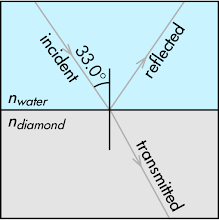## 20160215

### Physics quiz question: possible total internal reflection?

Physics 205B Quiz 1, spring semester 2016
Cuesta College, San Luis Obispo, CAA beam of light strikes the interface between room temperature water (index of refraction[*]1.333) and diamond (index of refraction 2.418), with an incident angle in water of 33.0°. (Drawing is not to scale.) If the incident angle of the beam of light in water is increased slightly from 33.0° to 33.5°, the incident beam would be:
(A) reflected back into water.
(B) transmitted into diamond.
(C) (Both of the above choices.)
(D) (Not enough information is given.)

[*] physics.info/refraction/.

Correct answer (highlight to unhide): (C)

Snell's law relates the indices of refraction with the incident and transmitted angles, where water is medium 1, and diamond is medium 2:

n1·sinθ1 = n2·sinθ2,

where the given (or assumed to be known) quantities, unknown quantities, and quantities to be explicitly solved for are denoted.

Directly solving for the transmitted angle (if any) in diamond:

sinθ2 = (n1/n2)·sinθ1,

θ2 = sin–1(n1/n2)·sinθ2) = sin–1((1.333/2.418)·sin(33.5°)) = 17.7144284003°,

or to three significant figures, the transmitted angle in diamond is 17.7°. Thus the light will be both reflected back into water and transmitted into diamond.

Student responses
Section 70856
Exam code: quiz01sN0w
(A) : 6 students
(B) : 6 students
(C) : 30 students
(D) : 1 student

Success level: 70%
Discrimination index (Aubrecht & Aubrecht, 1983): 0.43